Question

compute the value of current and the voltage of circuit in Figure 5. also obtain the energy stored in inductor and capacitor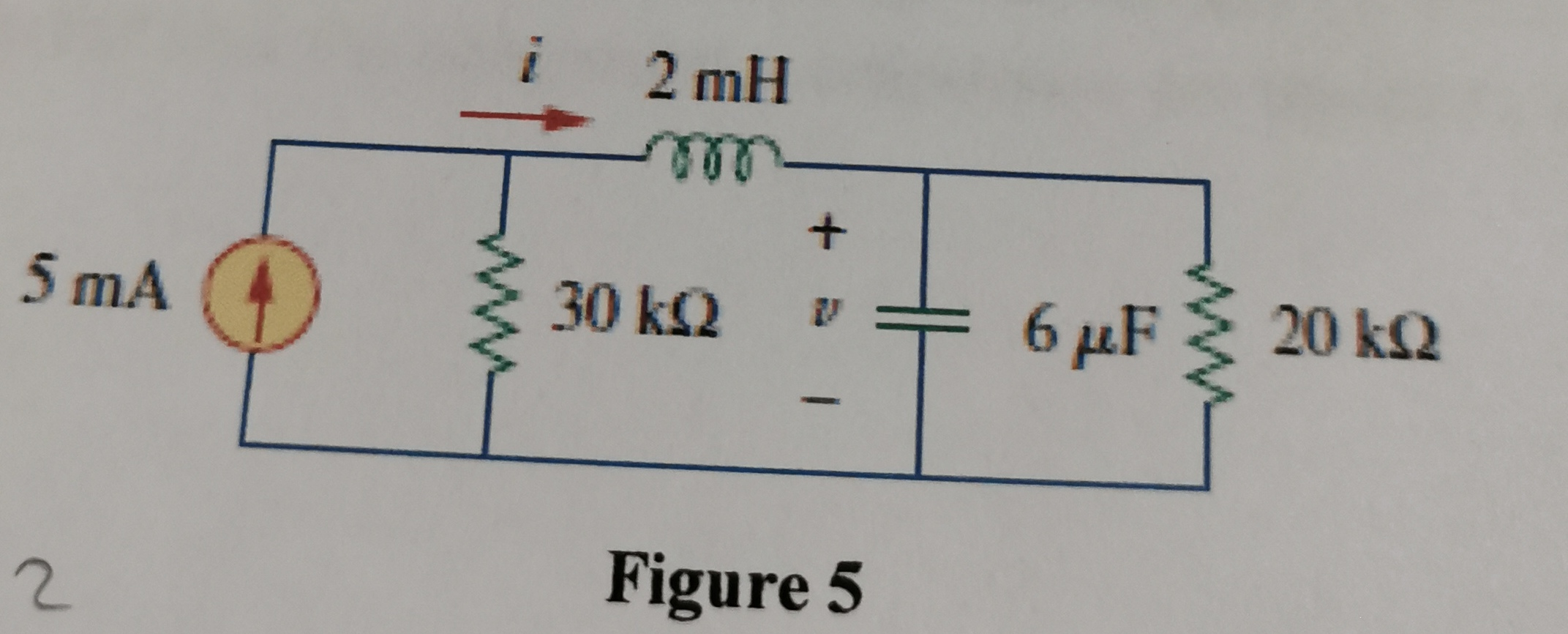We need at least 9 more requests to produce the answer.

1 / 10 have requested this problem solution

The more requests, the faster the answer.

All students who have requested the answer will be notified once they are available.

Earn Coins

Coins can be redeemed for fabulous gifts.

Similar Homework Help Questions
• A buck converter is operating in the steady state with an input voltage of = 42 V dc, D = 0.3, ou...

A buck converter is operating in the steady state with an input voltage of = 42 V dc, D = 0.3, output power of 24 W, an inductance of 25 H and a switching frequency of 400 kHz. Draw the input current, inductor current, inductor voltage, and capacitor current waveforms. Assume the dc component of the inductor current flows through to the load and the ac component of the inductor current flows through the capacitor. Assume ideal circuit components.

• Find the Steady State Voltage and Current Values. Develop the equation for i(t) , the current thr...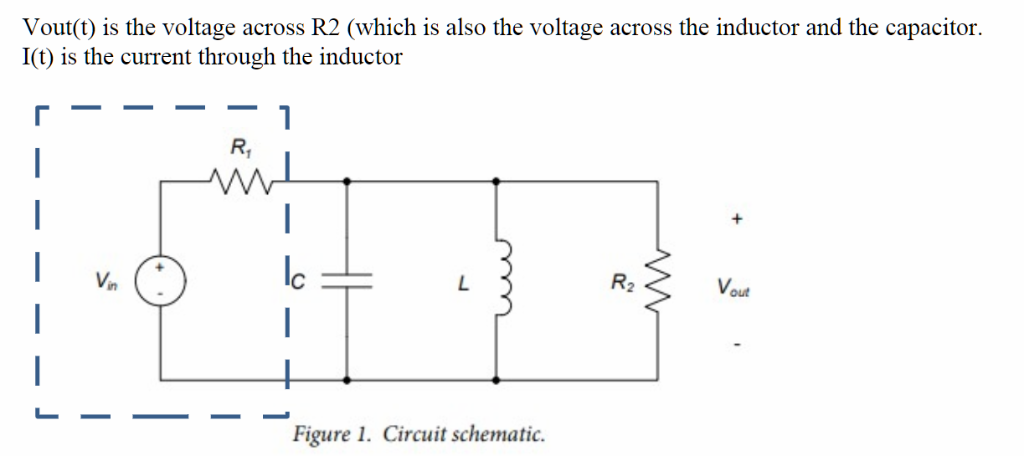Find the Steady State Voltage and Current Values. Develop the equation for i(t) , the current through the inductor and Vout(t). I need help, I don't know if my calculations are correct, I found the neper frequency to be: a=439.109 rad/sec and resonant frequency to be Wo=14586.5 rad/sec. This is a parallel step response RLC circuit The circuit is underdamped. Please show all work clearly so that I can understand the process. Vout(t) is the voltage across R2 (which is...

• Consider the series RLC circuit in Figure 1. Suppose the source voltage is initially OV, and...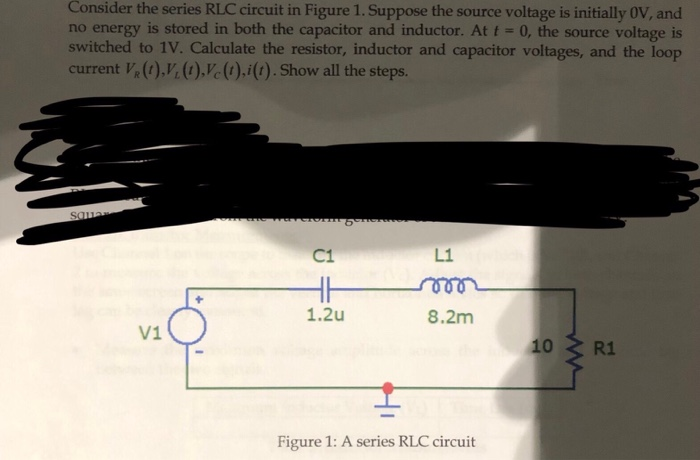Consider the series RLC circuit in Figure 1. Suppose the source voltage is initially OV, and no energy is stored in both the capacitor and inductor. At t = 0, the source voltage is switched to 1V. Calculate the resistor, inductor and capacitor voltages, and the loop current VROV.O.Vc),it). Show all the steps. SOL L1 n 8.2m 10 3 R1 Figure 1: A series RLC circuit

• 1. A 2.40 mH inductor is connected in series with a dc battery of negligible internal...

1. A 2.40 mH inductor is connected in series with a dc battery of negligible internal resistance, a 0.880 kΩ resistor, and an open switch. a. How long after the switch is closed will it take for the current in the circuit to reach half of its maximum value ? b. How long after the switch is closed will it take for the energy stored in the inductor to reach half of its maximum value? 2. A 4.75 μF capacitor...

• In the circuit to the right, the DC source, V, 2 volts for all time. The...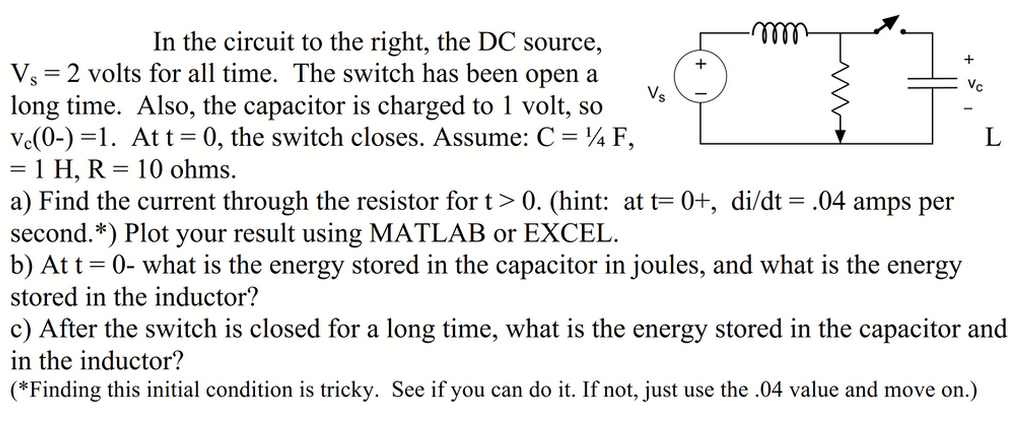In the circuit to the right, the DC source, V, 2 volts for all time. The switch has been open a long time. Also, the capacitor is charged to 1 volt, so vd0-) =1. At t-0, the switch closes. Assume: C ¼ F, - 1 H, R-10 ohms. a) Find the current through the resistor for t> 0. (hint: at t-0+, di/dt- .04 amps per second.*) Plot your result using MATLAB or EXCEL. b) Att -0- what is the energy...

• Consider the series RLC circuit in Figure 1. Suppose the source voltage is initially OV, and...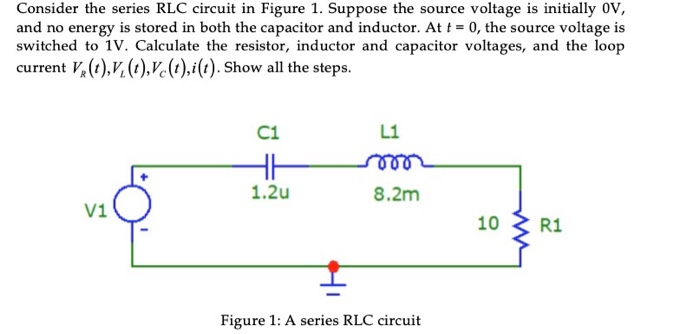Consider the series RLC circuit in Figure 1. Suppose the source voltage is initially OV, and no energy is stored in both the capacitor and inductor. At t = 0, the source voltage is switched to 1V. Calculate the resistor, inductor and capacitor voltages, and the loop current V (t),,(t),Vc(t),i(t). Show all the steps. C1 L1 1.2u 8.2m 10 3 R1 Figure 1: A series RLC circuit

• (a) Adjust the value of the 3 ? resistor in the circuit represented in Fig. 9.55...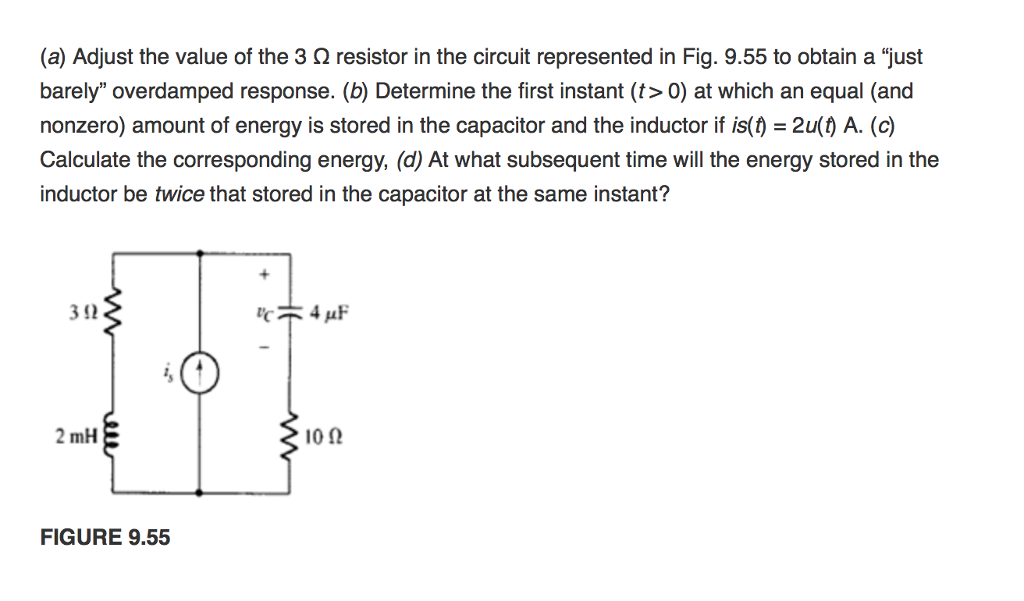(a) Adjust the value of the 3 ? resistor in the circuit represented in Fig. 9.55 to obtain a "just barely" overdamped response. (b) Determine the first instant (t> 0) at which an equal (and nonzero) amount of energy is stored in the capacitor and the inductor if is()-2u() A. (c) Calculate the corresponding energy, (d) At what subsequent time will the energy stored in the inductor be twice that stored in the capacitor at the same instant? 3 92...

• Problem 5 (20 points) No energy is stored in the 100 mH inductor or the 0.4...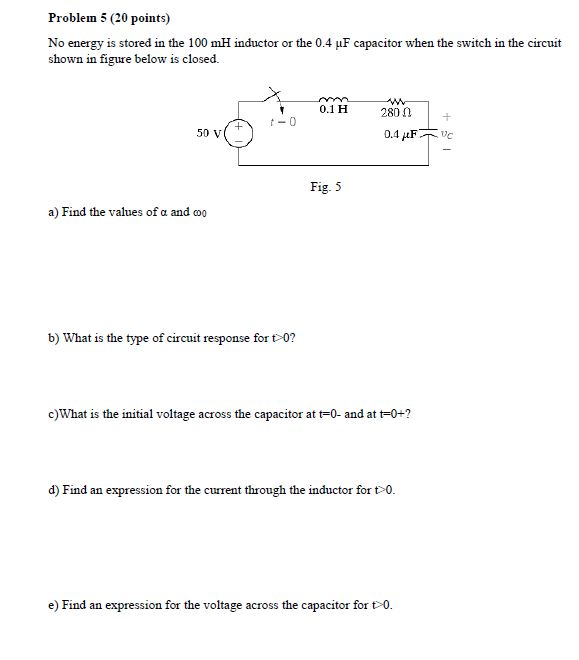Problem 5 (20 points) No energy is stored in the 100 mH inductor or the 0.4 LF capacitor when the switch in the circuit shown in figure below is closed. 0.1 H -O 2800 0.4 F 50 V uc Fig. 5 a) Find the values of a and co b) What is the type of circuit response for t>0? c)What is the initial voltage across the capacitor at t=0- and at t=0+? d) Find an expression for the current through...

• S2* (8 pts) In the circuit to the right, the DC source, V = 2 volts...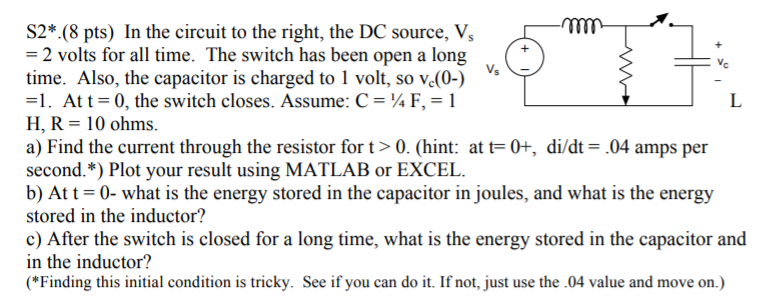S2* (8 pts) In the circuit to the right, the DC source, V = 2 volts for all time. The switch has been open a long time. Also, the capacitor is charged to 1 volt, so ve(0-) =1. At t = 0, the switch closes. Assume: C = "4 F, = 1 H, R = 10 ohms. a) Find the current through the resistor for t> 0. (hint: at t= 0+, di/dt = .04 amps per second. *) Plot your...

• An LC circuit like that in the figure below consists of a 3.30-H inductor and an...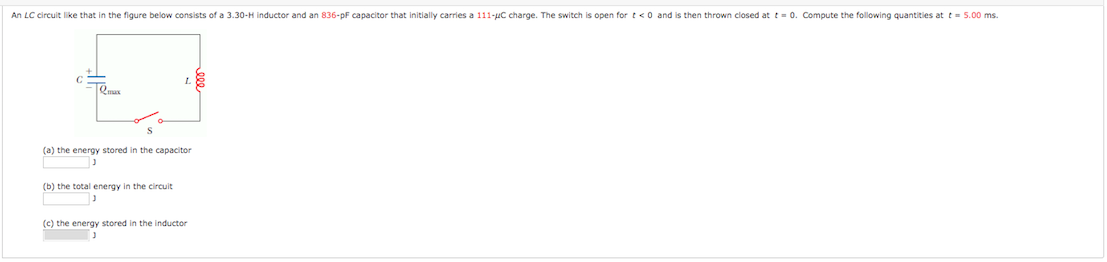An LC circuit like that in the figure below consists of a 3.30-H inductor and an 836-pF capacitor that initially carries a 111-C charge. The switch is open for <0 and is then thrown closed at t = 0. Compute the following quantities at t = 5.00 ms. L8 (a) the energy stored in the capacitor (b) the total energy in the circuit (c) the energy stored in the inductor